Kabir, S. M. M. (2023). “Process maximization of salt free reactive dyeing on cotton using Taguchi approach,” BioResources 18(3), 4543-4557.

#### Abstract

This study optimized the salt free reactive dyeing process using the Taguchi approach. Dyeing of cotton fabric with reactive dyes is popular because of its bright and brilliant color in various shade ranges. Cationization with ALBAFIX-WFF and the dyeing process on cotton fabric was carried out using the exhaust method. To determine the optimum process conditions, two types of multiple characteristic parameters, including the single characteristic value conversion method and the process maximization method, were used on the basis of color strength (K/S) and wash fastness. The single characteristic value conversion method confirmed that the optimum process condition was a cationization temperature of 40 °C and a dyeing pH of 11. Most importantly, the optimal conditions were confirmed by the process maximization method as a concentration of ALBAFIX-WFF 30 g/L, cationization temperature at 80 °C, dyeing pH 12, and material-to-liquor ratio (M:L) of 1:5.  More suitable dyeing properties are also achieved by the process maximization method.

Process Maximization of Salt Free Reactive Dyeing on Cotton using Taguchi Approach

Shekh Md. Mamun Kabir a,*

This study optimized the salt free reactive dyeing process using the Taguchi approach. Dyeing of cotton fabric with reactive dyes is popular because of its bright and brilliant color in various shade ranges. Cationization with ALBAFIX-WFF and the dyeing process on cotton fabric was carried out using the exhaust method. To determine the optimum process conditions, two types of multiple characteristic parameters, including the single characteristic value conversion method and the process maximization method, were used on the basis of color strength (K/S) and wash fastness. The single characteristic value conversion method confirmed that the optimum process condition was a cationization temperature of 40 °C and a dyeing pH of 11. Most importantly, the optimal conditions were confirmed by the process maximization method as a concentration of ALBAFIX-WFF 30 g/L, cationization temperature at 80 °C, dyeing pH 12, and material-to-liquor ratio (M:L) of 1:5. More suitable dyeing properties are also achieved by the process maximization method.

DOI: 10.15376/biores.18.3.4543-4557

Keywords: Reactive dyes; Salt free dyeing; Taguchi method; Color strength; Wash fastness

Contact information: Department of Wet Process Engineering, Bangladesh University of Textiles, Tejgaon, Dhaka-1208, Bangladesh; mamunkabir.butex@gmail.com

INTRODUCTION

Reactive dyes are the most popular dyestuffs used for cotton dyeing. A large amount of salt is required to achieve higher exhaustion of the reactive dye from the dye bath onto the fiber (Broadbent 2001). To overcome the repulsion forces occurring between the negative charged fibers and the dye molecules, electrolytes are needed for the dyeing process. However, the resulting discharge of salt has a harmful effect on the environment (Shore 2002). Recently, there has been improvement of the dye-ability on cotton fabric without salt. Pre-treatment with a cationic agent on cotton fabric has been studied as an alternative approach instead of using an electrolyte (salt) (Buschle-Diller and Zeronian 1992; Montazer 2007). Primary and secondary hydroxyl groups of cotton can actively participate in the chemical modification (Chattopadhyay et al. 2007; Montazer 2007; Choudhury 2014). Cotton fabric was cationized using CIBAFIX-WFF (a polyamino chlorohydrin quaternary ammonium compound) (Kannan 2006; Sanjit 2014). Salt-free reactive dyeing was carried out by cationization of cotton (Hauser and Tabba 2001; EI-Shishtawy and Nassar 2002; Montazer 2007) and Ramie fiber with 3-chloro-2-hydroxypropyltrimethylammonium chloride (CHPTAC) (Liu et al. 2007). In addition, cationization of jute fabrics by ALBAFIX-WFF (poly-diallyl dimethyl ammonium chloride) and sodium hydroxide was found to improve the dye-ability of reactive dye (Arju et al. 2014). The cationization of cotton fabric using chitosan (Bhuiyan et al. 2014), Kemifix REA, Optifix F, and Optifix RSL (Mustafa Tutak et al. 2010), Solfix-E (polyaminochlorohydrin quaternary ammonium salt with epoxide functionality), and polyacryloxyethyltrimethylammonium chloride (PAOTAC) was used as a cationic agent for salt-free reactive dyeing (Lewis and McIlroy 1997; Teng et al. 2011). The cationized cotton substrate would be a suitable starting material of eco-friendly dyeing process for reducing the pollution load in terms of salinity and color discharge from the textile dyeing industries (Khatri et al. 2015; Arivithamani and Giri Dev 2017). However, the addition of NaOH for cationization caused the pH to rise to a very high level; subsequent neutralizing of the pH in wastewater treatments results in in-situ production of salt (Novak et al. 1998).

The Taguchi method was used to find out the optimum condition for Digital Textile Printing Process (Jung et al. 2016). The Taguchi method was used to find sewing conditions that minimize the seam pucker (Park and Young 2005) and maximize the delamination strength of fusible interlinings (Yoon et al. 2010). Dyeing process optimization and color strength prediction for viscose/Lycra blended knitted fabrics was measured by the Taguchi Method (Hossain et al. 2016). Optimization of the dyeing process of cotton knit fabric and reduction of the re-dyeing process was analyzed by the Taguchi method and ANOVA (Analysis of variance) (Wahyudin et al. 2017). Optimization of chemical coagulation of real textile wastewater was investigated by the Taguchi method (Gokkus et al. 2012; Swapnil et al. 2015). Kuo and Lin (2019) used the Taguchi method and fuzzy theory to find optimum processing parameters for sueding fabric comfort. Investigating the effect of temperature, heating time, concentration, and particle size on the improved gel spinning process of ultra-high molecular weight polyethylene (UHMWPE) was measured using the Taguchi method (Rajput et al. 2018). Analysis for optimization of coating process conditions for denim fabrics (Üstüntağ et al. 2020), the bursting strength of knitted fabrics (Mavruz and Ogulata 2010), and optimization of concrete strengthened with polymer after high temperature (Mavruz and Ogulata 2010) were also studied by the Taguchi method. The Taguchi method was used for design optimization of cutting parameters (Yang et al. 1998) and optimization of end milling parameters (Ghani et al. 2004).

The present studies were related to cotton fabric treated with cationizing agent in order to enhance the use of salt-free for reactive dyeing. The resulting performance was evaluated by color strength, exhaustion, fixation, and fastness properties. In the present optimized system, it might be assumed that NaOH is playing the role in increasing the ionic strength of the aqueous system as a means of suppressing electrostatic repulsions. However, there has been no research conduct to analyze the optimum condition by the help of Taguchi approach based on two characteristics, such as color strength and wash fastness. The multiple characteristic values are converted by statistical analysis into a single characteristic value and process maximization method. In addition, the most suitable statistical value was analyzed by the performance of dyeing properties.

EXPERIMENTAL

Materials

Cotton (1 × 1 single jersey, GSM 150, scoured and bleached) fabric was used for the experiment. AlBAFIX-WFF (supplied by HUNTSMAN) was applied as a cationizing agent for cationization of cotton fabric. Novacron Ruby S-3B (supplied by HUNTSMAN) reactive dye (2% shade) was used for dyeing the fabric.

Cationization

Cotton fabric was treated with ALBAFIX-WFF (20/30/40 g/L) along with NaOH (20 g/L) at a temperature of 40 °C/60 °C/80 °C for 20 min. The material-to-liquor ratio (M:L) (w:v) ratio was taken as 1:10, and the treatment was carried out in exhaust method. After cationization, fabric was washed and dried in open air for 24 h.

Dyeing

Cotton fabric was dyed with 2% (owf) Reactive dye (Novacron Ruby S-3B), 2 g/L wetting agent, 1 g/L leveling agent, and the required amount of soda wash (Na2CO3) to maintain the specified pH. Dyeing was carried out in the exhaust method at 60 °C for 60 min. The pH was set to either10, 11, or 12, and the M:L ratio (w:v) was 1:5, 1:10, and 1:15, respectively. After dyeing, the fabric was washed with cold water (room temperature) then hot water (70 °C) and again cold water. Finally, the sample was dried in an oven dryer.

Measurement of Color

The color strength (k/s) values of the dyed cotton fabrics were analyzed using a spectrophotometer (Datacolor 650, USA, standard light D65; 10o standard observer, specular component included). The color strength (k/s) value was calculated from the sample reflectivity (R), as follows,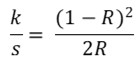(1)

where k is the absorption coefficient, s is the scattering coefficient, and R is the reflectivity (McDonald et al 1997).

Measurement of Color Fastness

Color fastness to wash was evaluated according to ISO 105-C06 (A2S). In addition, color staining was assessed with the help of Grey Scale and Light Box (D65 light source).

Experimental Design

Several factors are known to affect the color strength (K/S) value and wash fastness. Four important controllable factors were chosen in this study: ALBAFIX-WFF (A), cationization temperature (B), dyeing pH (C), and material and liquor ratio (M:L) (w:v) of dyeing (D). Their levels are as shown in Table 1. The orthogonal array selection rules are given in Table 2. The L9 (34) orthogonal array is shown in Table 3. Each experiment was repeated three times.

Table 1. Different Factors and Levels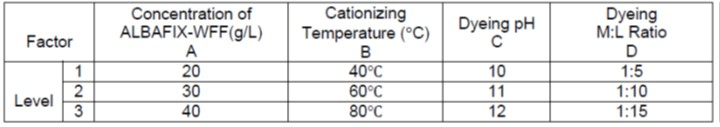Table 2. Selection Process of Orthogonal Array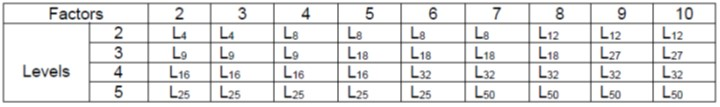Table 3. L9 (34) Table of Orthogonal Array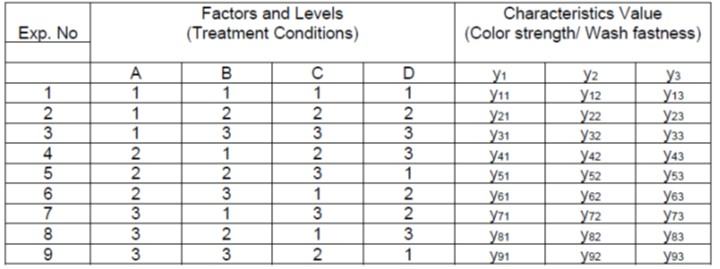Both color strength (k/s) and wash fastness values were determined. The signal to noise (S/N) ratio of each experiment was calculated using Eq. 2 (Jung et al. 2016).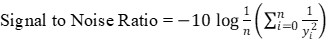(2)

The number of repetitions for an experimental combination is n, the index number is i, and yi is the characteristic value. In the Taguchi method, the S/N ratio is equivalent to the inverse of expected loss. Therefore, the expected loss is proportional to the S/N ratio. In this study, color strength (k/s) and wash fastness were chosen for characteristic value y and 9 S/N ratios were calculated using three characteristic values and measured three times per interval.

Process Evaluation by Dye Exhaustion, Fixation (%) and Levelness Parameter

The maximum process method was evaluated by dye exhaustion and fixation (%), which was determined by UV-Vis spectrophotometry (Cintra 2020, GBC, Australia). The percentage dye exhaustion (E%) and fixation (F%) were calculated according to the following equations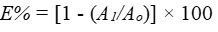(3)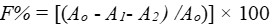(4)

Here, Ao and A1 are the absorbance of the dye solution at λmax before and after dyeing. A2 is the absorbance of the dye-soaped solution with a non-ionic surfactant. The levelness of the dyed fabric was assessed using an instrumental method that was developed by Yang and Li (1993).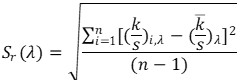(5)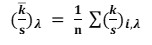(6)

Here, λ is the wavelength for the measurement, n is the total number of measurements, and (k/s)i,λ is the k/s value of the ith measurement at λ.

The levelness parameter was modified by Koh et al. (2001). Here, Sr(λ) is the relative sample standard deviation of (k/s)λ and V(λ) is the spectral luminous function. Thus, the unlevelness value is described as: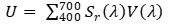(7)

The levelness parameter LLev is varied to give different values, which are very similar to the gray-scale rating for color change if (U0.3114)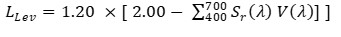(8)

And if U< 0.3114, then: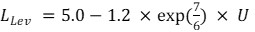(9)

RESULTS AND DISCUSSION

Optimum Condition by Taguchi Method

Color strength (k/s) and wash fastness were taken as characteristics values. Four factors and three levels were considered to find optimum conditions based on the two characteristic values of color strength (k/s) and wash fastness. In this study, optimum conditions were calculated by two methods, i.e. by means of single characteristics and when using the process maximization selection method.

Single Characteristics data Conversion Method

The average characteristic values and S/N ratios are shown in Tables 4, 5, and 6.

Table 4. Normalized Characteristics Value for Color Strength (k/s)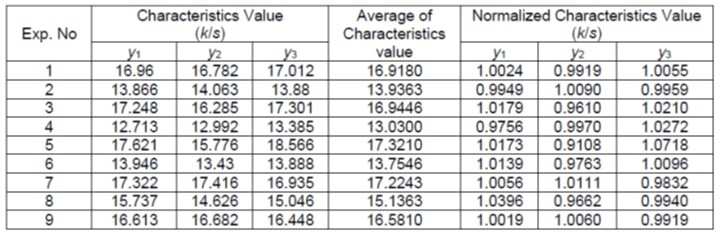Normalized characteristics value was calculated using Eq. 10 (Jung et al. 2016).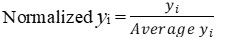(10)

Likewise, the signal to noise ratio was calculated as follows: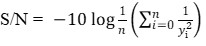(11)

Here, the number of repetitions for a multiple experiment is n, the index number is i, and yi is the characteristic value of the ith experiment.

Table 5. Normalized Characteristics Value for Wash Fastness (Staining on Cotton)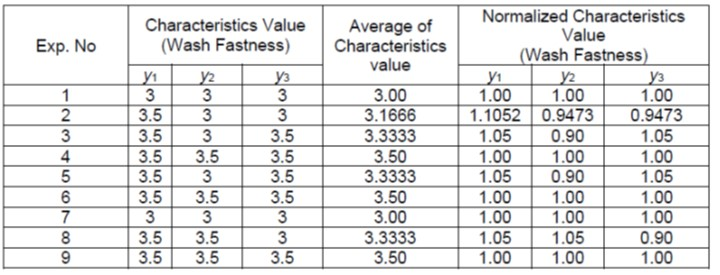Table 6. S/N Ratio of Average Characteristics Value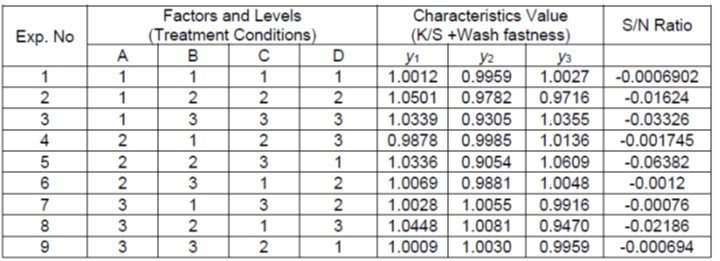The sum of squares was calculated using Eq. 11.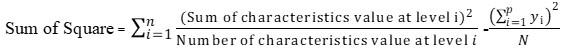(11)

where yi is the characteristic value at level i, p is the number of levels, and N is the total number of characteristic values. The S/N Ratio was calculated using Eq. 12.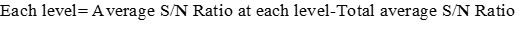(12)

The total average S/N Ratio was calculated using Eq. 13.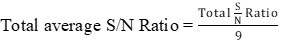(13)

The results of the analysis of the S/N ratio are shown in Table 7.

Table 7. Analysis of S/N Ratio for Single Characteristic Value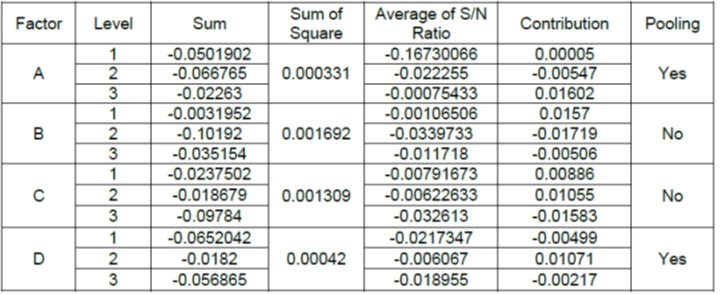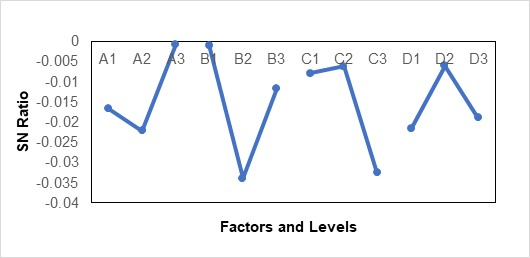Fig. 1. Cause and effect diagram of single characteristic value for color strength and wash fastness

Factors B and C had the largest values of 0.001692 and 0.001309 by sum of squares. Factors A and D, with relatively small sum of square error, were pooled for error. The results of the F-Test are shown in Table 8. The degrees of freedom were calculated using Eq. 14.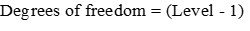(14)

Table 8. ANOVA Results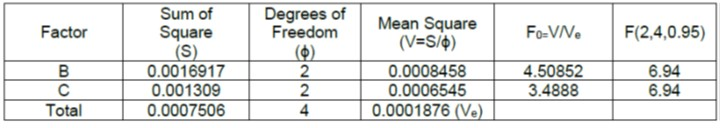Cationization temperature and dyeing pH were considered to be the factors affecting color strength (k/s) and wash fastness. The optimum condition was B1 C2 where the S/N Ratio of each factors became the highest, with a cationization temperature of 40 ℃ and dyeing at pH 11.

Average and Confidence Interval under Optimum Condition:

The optimal S/N Ratio (SNo) can be predicted as follows,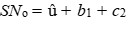(15)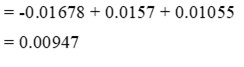where û is the average S/N ratio, b1 is the contribution of B1, and c2 is the contribution of C2.

Difference of Expected Loss

The current condition was experiment 2 in Table-VIII and its S/N ratio (SNc) was -0.01624. The predicted S/N ratio at maximum condition (SNo) was 0.00947. The difference of expected losses can be done using equation (16).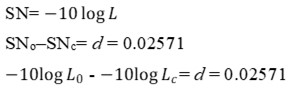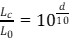(16)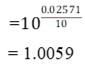where L is the expected loss of characteristics value and it means the variance of characteristics value. In this case, the expected loss is 1.0059 times larger than that of optimum condition, which means that color strength (K/S) and fastness have been improved.

Process Maximization Method

Single parameter design for each characteristic value

The optimum two characteristic values have been chosen according to the single parameter design method.

Color Strength Results

S/N Ratio of Color Strength (k/s) are as represented in Table 9.

Table 9. S/N Ratio (k/s value) for Process Maximization Method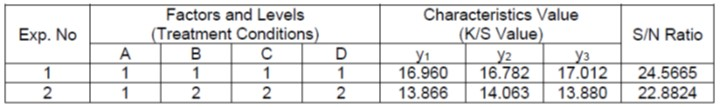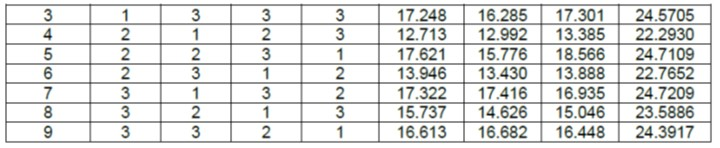Table 10. Evaluation of S/N Ratio (K/S Value) for Process Maximization Method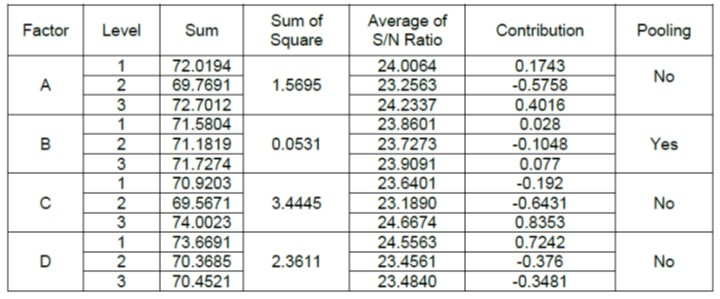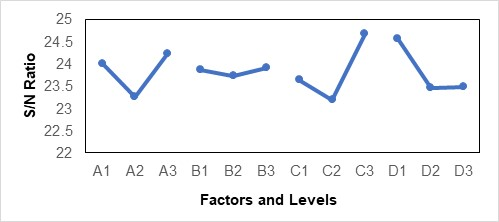Fig. 2. Cause and effect diagram of process maximization value for color strength and wash fastness

The sum of square of factors A, C, and D had the largest value of 1.5695, 3.4445, and 2.3611, respectively. F-tests are shown in Table 11.

Table 11. ANOVA Results (K/S value)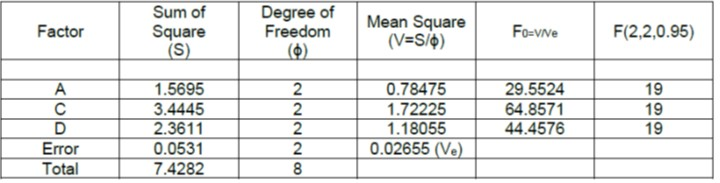Table 17 factors A, C and D could be considered to be meaningful. Concentration of ALBAFIX-WFF, dyeing pH and M: L Ratio considered to be the factors affecting highest color strength (K/S) and wash fastness. The optimum condition was A3 C3 D1 where S/N ratio of each factors becomes largest, such as Concentration of ALBAFIX-WFF 40 g/l, Dyeing pH of 12 and M: L Ratio of 1:5.

Wash Fastness Results

S/N Ratio for Wash Fastness are illustrated in Table 12.

Table 12. S/N Ratio (Wash Fastness)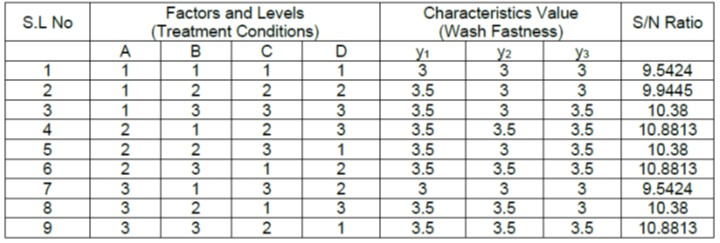Table 13. Results of S/N Ratio (Wash Fastness)